## Angular Momentum Algebra: Raising and Lowering Operators

We have already derived the commutators of the angular momentum operatorsWe have shown that angular momentum is quantized for a rotor with a single angular variable. To progress toward the possible quantization of angular momentum variables in 3D, we define the operatorand its Hermitian conjugate.Since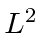commutes with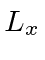and, it commutes with these operators.The commutator withis.From the commutatorsand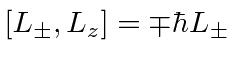, we can derive the effect of the operators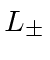on the eigenstates, and in so doing, show thatis an integer greater than or equal to 0, and thatis also an integer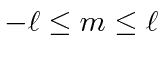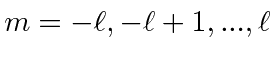Therefore,raises thecomponent of angular momentum by one unit of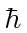andlowers it by one unit. The raising stops when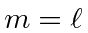and the operation gives zero,. Similarly, the lowering stops because.Angular momentum is quantized. Any measurement of a component of angular momentum will give some integer times. Any measurement of the total angular momentum gives the somewhat curious resultwhereis an integer.

Note that we can easily write the components of angular momentum in terms of the raising and lowering operators.We will also find the following equations useful (and easy to compute).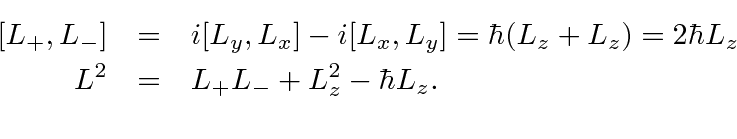* Example: What is the expectation value ofin the state?*

* Example: What is the expectation value ofin the state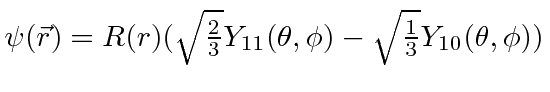?*

Jim Branson 2013-04-22